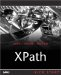Creating Logical Expressions

 A logical expression uses logical operators and is a compound expression. Unless there's an error, its value is always either true or false . Here are the logical operators: and Performs a logical and operation or Performs a logical or operation The and operator lets you connect two Boolean operands and returns true if both operands are true, and false otherwise . The or operator lets you connect two logical operands and returns true if either operand is true, and false otherwise. Here's an example. Say that \$temperature holds a value of 72; in that case, this example returns a value of true : \$temperature < 80 and \$temperature > 60 And this expression also returns true , because one logical operand ( \$temperature > 60 ) is true: \$temperature > 80 or \$temperature > 60 THE not FUNCTION XPath 2.0 also has a function named not() , which reverses the Boolean value of the value you pass to it. For example, if \$temperature holds a value of 72, then \$temperature = 72 is true, and not(\$temperature = 72) is false. More on this function is coming up in Chapter 11, "XPath 2.0 Boolean, QName, and Date Functions." This expression returns false : \$temperature > 80 and \$temperature > 60XPath Kick Start: Navigating XML with XPath 1.0 and 2.0
ISBN: 0672324113
EAN: 2147483647
Year: 2002
Pages: 131

Similar book on Amazon